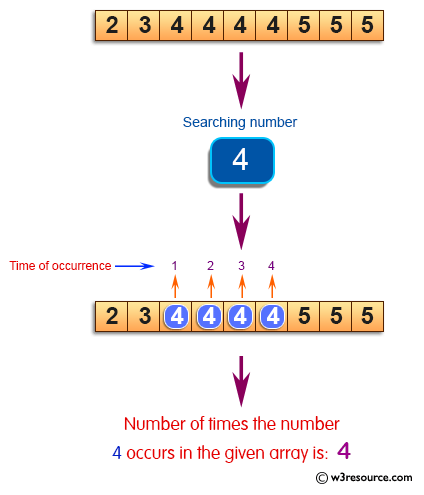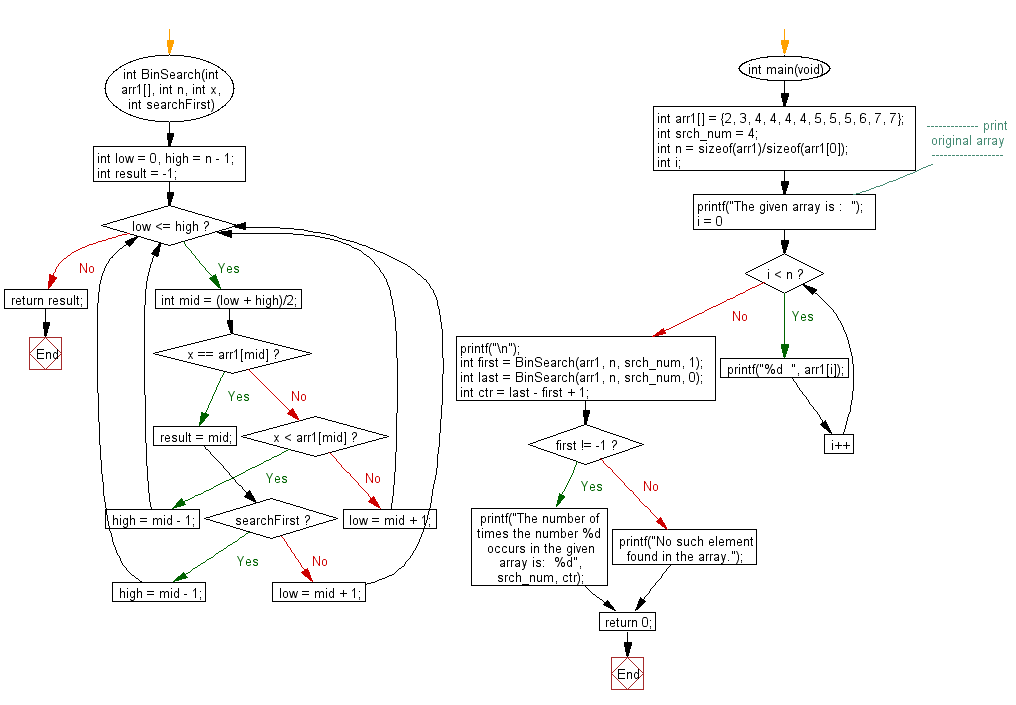﻿ C exercises: Find the number of times occurs a given number in an array - w3resource# C Exercises: Find the number of times occurs a given number in an array

## C Array: Exercise-53 with Solution

Write a program in C to find the number of times (frequency) occurs a given number in an array.

Pictorial Presentation:Sample Solution:

C Code:

``````#include <stdio.h>
int BinSearch(int arr1[], int n, int x, int searchFirst)
{
int low = 0, high = n - 1;
int result = -1;
while (low <= high)
{
int mid = (low + high)/2;
if (x == arr1[mid])
{
result = mid;
if (searchFirst)
high = mid - 1;
else
low = mid + 1;
}
else if (x < arr1[mid])
high = mid - 1;
else
low = mid + 1;
}
return result;
}

int main(void)
{
int arr1[] = {2, 3, 4, 4, 4, 4, 5, 5, 5, 6, 7, 7};
int srch_num = 4;
int n = sizeof(arr1)/sizeof(arr1);
int i;
//------------- print original array ------------------
printf("The given array is :  ");
for(i = 0; i < n; i++)
{
printf("%d  ", arr1[i]);
}
printf("\n");
//------------------------------------------------------
int first = BinSearch(arr1, n, srch_num, 1);
int last = BinSearch(arr1, n, srch_num, 0);
int ctr = last - first + 1;
if (first != -1)
printf("The number of times the number %d occurs in the given array is:  %d", srch_num, ctr);
else
printf("No such element found in the array.");
return 0;
}```
```

Sample Output:

```The given array is :  2  3  4  4  4  4  5  5  5  6  7  7
The number of times the number 4 occurs in the given array is:  4
```

Flowchart:C Programming Code Editor:

Improve this sample solution and post your code through Disqus.

What is the difficulty level of this exercise?

﻿